# The Beautiful Mathematics Behind the RSA Cryptosystem

## What is RSA?

RSA or Rivest-Shamir-Adleman is a public-key cryptosystem that was first described in 1977 by Ron Rivest, Adi Shamir and Leonard Adleman. RSA is one of the oldest public-key cryptosystem but not the first. The first ever publicly known system was the Diffie-Hellman key exchange published just one year prior to RSA.

### Asymmetric Cryptography

Asymmetric cryptography or public-key cryptography is a type of cryptography that uses a pair of keys.

• Public key
• Can be known by others
• Used to encrypt data
• Private key
• Only known by the end user
• Used to decrypt, public key encrypted data

Asymmetric cryptography is most popularly used in SSH (secure shell) to verify identity prior to exchanging secret key to be used for symmetrical cryptographic communications.

## How does RSA work?

Warning this section contains a lot of math, the math concepts are that of an engineer who has zero background of number theory and learned on the spot. Concepts are explains to the best of my understanding and links to specific top articles are provided for people who want to dive deeper.

### Trapdoor Function

A trapdoor function is a function that is easy to compute in one direction, yet very difficult to do in the backward direction. For example:

Given the equation find x and y, given that both are prime.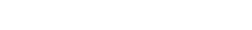To solve for x and y one would need to divide 4,284,689 by several prime numbers until an answer is found, essentially using brute force. But if given 1,171 as p, one could easily divide 4,284,689 by 1,171 and get 3,659 as q.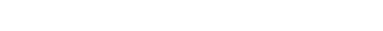This would be a good example of a trap door function for a human but not one for a computer who could brute force all the combinations of prime products under a second.

To prevent brute forcing, RSA uses very large prime numbers. The default length for ssh-keygen is 3072-bit, or a number that is 925 digits long.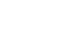### Prime and Modulus Generation

The first step to generating a RSA key pair is to generate two prime numbers, q and p. This can be done using a primality test algorithm. The algorithm may look like this:

1. Generate a list of primes in a range of values via a prime sieve function, such as the sieve of Eratosthenes.
2. Filter the list of primes for Sophie-Germain primes candidates, this generates a list of “safe primes”.
3. The above list is checked against small known primes of less then 230 , those are then filtered out to provide a list of candidates.
4. Take the list of candidates and perform the Miller-Rabin primality test with a minimum of 4 trials, this generates a list of validated “safe primes”.
5. Select two primes from the validated list of “safe” prime and multiply them together, this will be the modulus. Used in the encryption and decryption key. We will call this number n. For our case we can select p=11, n=13The above algorithm is summarized based off the the open-ssh’s implementation of ssh-keygen found here.

### Find Number of Relatively Prime (coprime) Numbers

Integers are coprime when the only possible integer divisor of both of them is 1.

For example 14 and 25 are coprime because 14 and 25 share no common divisor other than 1. An easy way to thing about this is to put them into a fraction. If the fraction can be reduced then the two numbers are not coprime to each other.There are two functions that can be used to calculate the number of coprimes from 1 to n. Euler’s totient function and Carmichael’s totient function.

#### Euler’s Totient Function

Euler’s totient function counts the number of positive integers up to a given integer n that is relatively prime to n. This number is represented as the greek letter phi φ(n).

An easy way to calculate φ(n) is:#### Carmichael’s Totient Function

Carmichael’s Totient Function is similar to Euler’s function except it is abled to be reduced by half under certain circumstances. This number is represented as the greek letter lambda λ(n). I won’t go into detail on his exact theorem, you can click on the link to find out more. But here is an online calculator.If needed to be calculated programmatically, Carmichael’s Totient Function is usually used and will use less resources to calculate all the prime factors, due to the smaller λ(n) in relation to φ(n) in most cases.

### Generating the Public Key

Now that we have φ(n) or λ(n) we can construct our public key. A public key is made up of a prime number e, as well as a modulus n. For our case we will use λ(n) as our coprime count.

The criteria for e is that it must fall between 1 and λ(n), which in our example is 6 and it must be coprime with n, which is 14 and λ(n). In our case the only one left is 5, so thus e is 5. We can write our encryption formula applying our public key and a plaintext message m with the following formula:### Encrypting a Message

In the above example e mod n is the public key. Mod refers to modulus or the left over during division by a divisor. If want to encrypt a single number 4 and keep it secret. We can say that our plaintext, m, is equal to 4. Plugging everything in we get:To get the modulo I used wolframalpha, a convenient computational knowledge engine. After calculating the equation we get our encrypted value of 2. We can safely send this to the owner of the key pair, who should be the only person that can decrypt it. When decrypted the message should resolve to our initial plaintext value of 4.

Since the public key is made public, it is not important for e to be a random number. In the default key generation for ssh-keygen this value is set at 65,537. In order to make this possible the two prime numbers generated who’s product are n, must have 65,537 (Fermat number) coprimes or greater. This is why RSA keys have a minimum number of bits, which usually create a modulus much greater then the Fermat number, the selected numbers are then tested to ensure that 65,537 is coprime with n and λ(n), if it is not another set is selected.

### Generating the Private Key

In order to decrypt the encrypted message and turn it back into plaintext, we must create the private key. The creation of this key is pretty easy if you have all the required variables, which we luckily calculated out in the steps above. The first step we need to do is calculate d, given the formula below, which describes the criteria for selecting d.In plain english what we are looking for in the equation below is, what multiplied by 5 divided by 6 has a remainder of 1 (think back to your long division days.

We begin by finding out the numbers that are multiples by 5 that when divided by 6 give a remainder of 1, we can do this by listing out the multiples of 6 and the multiples of both 5 and 6. The 6 column is offset by 1 because we want to find the smallest multiple of 6 that can go into a multiple of 5, or else we would have no remainder..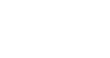We can see that we get two numbers 5, and 11. We can’t pick 5 because our encryption key is 5, so that leaves 11 left for our decryption key.

Now that we have a value of 11 for d we can create our decryption formula, by substituting in our private key.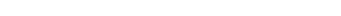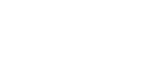### Decrypting A Message

Now to substitute our encrypted text value of 2.Again with the help of wolframalpha we were able to solve the equation above and get a decrypted value of 4. Which is consistent with our input plaintext value of 4.

A great online resource by Syed Umar Anis that does all the calculations can be found here. All you need to do is enter in two prime numbers.

## Final Thoughts

I hope this post helped you understand RSA as much as it did for me writing it. Now you understand what happens when you generate an RSA key and how it is used by both the client and the host during a ssh session.

### Related Posts## Terraform Tips & Tricks – Part 1 – Building A Constant Reference

One of the most common problems I see in large organizations when working with terraform is consistency. When we have a large amount of resources being managed…## Everything You Ever Wanted to Know About Istio but Were Afraid to Ask

Istio is a powerful service mesh that integrates natively with Kubernetes, I have been using Istio as my service mesh, ingress, and egress gateways on my personal…## How to Monitor Your Enphase Home Solar System with Telegraf

How to collect metrics from an Enphase Envoy PV system, with telegraf and influxdb.## How to Deploy Anthos on Bare Metal On-Prem

Introduction The main advantage of Anthos on BM over Anthos on VMWare for on-prem deployments is the ability to run Anthos clusters without a hypervisor license. Cluster…## OPA Gatekeeper: Bringing Law and Order to Kubernetes

Introduction Open Policy Agent (OPA) is a policy based control agent that is able to be integrated on various platforms. For the sake of this document we…## How to Setup Anthos on GKE Autopilot with Private Certificate Authority

What You Will Create The guide will set up the following: 2 Private GKE autopilot clusters with master global access ASM with multicluster mesh IstioIngress gateway to…